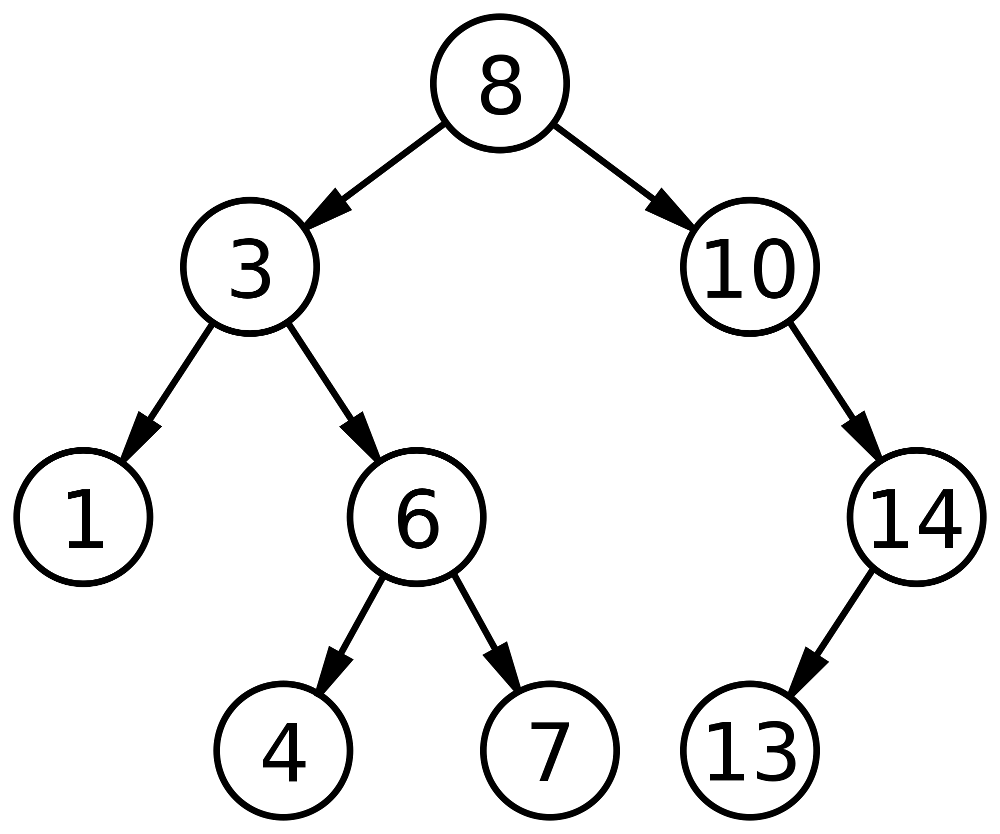# Solution to TUCTF 2017 Future taskFuture

reverse

250

#### Writeup

We received a small c program that implemented a simple hash funktion.

In the program was a string that represented a hashed password and a simple hash implementation, our task was to find out what input string hashed into the byte array named pass.

The hash algorithm consisted of two steps.

• A reordering of the original input string into a array in memory. Implemented in the function genMatrix.
• The creation of the hashed by adding different bytes from the original string together. Implemented in the function genAuthString.

This was the source code of the given problem:

``````#include <stdio.h>
#include <string.h>
#include <stdlib.h>

void genMatrix(char mat, char str[]) {
for (int i = 0; i < 25; i++) {
int m = (i * 2) % 25;
int f = (i * 7) % 25;
mat[m/5][m%5] = str[f];
}
}

void genAuthString(char mat, char auth[]) {
auth = mat + mat;
auth = mat + mat;
auth = mat + mat;
auth = mat + mat;
auth = mat + mat;
auth = mat + mat;
auth = mat + mat;
auth = mat + mat + mat;
auth = mat + mat + mat;
auth = mat + mat;
auth = mat + mat;
auth = mat + mat;
auth = mat + mat;
auth = mat + mat;
auth = mat + mat;
auth = mat + mat;
auth = mat + mat;
auth = mat + mat;
}

int main() {
char flag;
printf("What's the flag: ");
scanf("%25s", flag);
flag = 0;

if (strlen(flag) != 25) {
puts("Try harder.");
return 0;
}

// Setup matrix
char mat;// Matrix for a jumbled string
genMatrix(mat, flag);
// Generate auth string
char auth; // The auth string they generate
auth = 0; // null byte
genAuthString(mat, auth);
char pass = "\x8b\xce\xb0\x89\x7b\xb0\xb0\xee\xbf\x92\x65\x9d\x9a\x99\x99\x94\xad\xe4\x00";

// Check the input
if (!strcmp(pass, auth)) {
puts("Yup thats the flag!");
} else {
puts("Nope. Try again.");
}

return 0;
}
``````

Looking at the genAuthString method, we noticed that all but two of the equations only added two bytes, and we guessed that the flag only contained ascii characters. Since two ascii characters added are never more than 256 we didn’t have to worry about overflows for those, and if we got an overflow on the bytes with 3 values then we could handle that manually.

This meant that the hashing algorithm could be represented as a system of linear equations, and we knew that the flag was on the format TUCTF{…} so we had a seven characters of plain text.

We wrote a small program that printed the equations:

``````#include <stdio.h>
#include <string.h>
#include <stdlib.h>

void genMatrix(char mat, char str[]) {
for (int i = 0; i < 25; i++) {
int m = (i * 2) % 25;
int f = (i * 7) % 25;
mat[m/5][m%5] = str[f];
}
}

void printEquations(char mat, unsigned char auth[]) {
printf("%c + %c = %u\n", mat, mat, auth);
printf("%c + %c = %u\n", mat, mat, auth);
printf("%c + %c = %u\n", mat, mat, auth);
printf("%c + %c = %u\n", mat, mat, auth);
printf("%c + %c = %u\n", mat, mat, auth);
printf("%c + %c = %u\n", mat, mat, auth);
printf("%c + %c = %u\n", mat, mat, auth);
printf("%c + %c + %c = %u\n", mat, mat, mat, auth);
printf("%c + %c + %c = %u\n", mat, mat, mat, auth);
printf("%c + %c = %u\n", mat, mat, auth);
printf("%c + %c = %u\n", mat, mat, auth);
printf("%c + %c = %u\n", mat, mat, auth);
printf("%c + %c = %u\n", mat, mat, auth);
printf("%c + %c = %u\n", mat, mat, auth);
printf("%c + %c = %u\n", mat, mat, auth);
printf("%c + %c = %u\n", mat, mat, auth);
printf("%c + %c = %u\n", mat, mat, auth);
printf("%c + %c = %u\n", mat, mat, auth);
printf("a = 84\n");
printf("b = 85\n");
printf("c = 67\n");
printf("d = 84\n");
printf("e = 70\n");
printf("f = 123\n");
printf("y = 124\n");
}

int main() {
char* flag = "abcdefghijklmnopqrstuvwxy";
char mat;// Matrix for a jumbled string
unsigned char pass = "\x8b\xce\xb0\x89\x7b\xb0\xb0\xee\xbf\x92\x65\x9d\x9a\x99\x99\x94\xad\xe4\x00";
genMatrix(mat, flag);
printEquations(mat, pass);
}
``````

After we had the equation system we just used this solver: https://quickmath.com/webMathematica3/quickmath/equations/solve/advanced.jsp

And got these results: a=84 b=85 c=67 d=84 e=70 f=123 g=53 h=121 i=53 j=55 k=t1 l=109 m=53 n=94 o=48 p=101 q=95 r=52 s=95 t=100 u=48 v=119 w=111 x=33 y=124

which translates to TUCTF{5y573m5_0f_4_d0wn!}